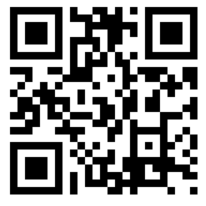# QR code generation using API GoogleFor generation the API from Google is used (http://chart.apis.google.com/chart). By creating the necessary URL, it is possible to download a picture generated using API. It is made without the use of external components in order that it was guaranteed to work in the web-client.

So, what does the approach consist:

1. Generate the text to be written in the qr-code.
3. Place the picture in our spreadsheet document.

Code:

```&AtServer
Function Hex(Val Value)

Value=Number(Value);
If Value<=0 Then
Result="0";
Else
Value=Int(Value);
Result="";
While Value>0 Do
Result=Mid("0123456789ABCDEFGHIJKLMNOPQRSTUVWXYZ", Value%16 + 1,1)+Result;
Value=Int(Value/16) ;
EndDo;
EndIf;

If StrLen(Result) < 2 Then
Result = "0" + Result;
EndIf;

Return "%" + Result;

EndFunction

&AtServer
Function EncodeURL(URL)
Rez = "";
For MF = 1 To StrLen(URL) Do
Ch = Mid(URL,MF,1);
Vch = CharCode(Ch);

If ("A" <= Ch ) And ( Ch <= "Z") Then      // "A".."Z"
Rez = Rez + Ch;
ElsIf ("a" <= Ch ) And ( Ch <= "z") Then // "a".."z"
Rez = Rez + Ch;
ElsIf ("0" <= Ch ) And ( Ch <= "9") Then // "0".."9"
Rez = Rez + Ch;
ElsIf (Ch = " ") Or ( Ch = "+") Then          // space
Rez = Rez + "+";
ElsIf (Ch = "-" ) Or ( Ch = "_")       // unreserved
Or (Ch = ".") Or (Ch = "!")
Or (Ch = "~") Or (Ch = "*")
Or (Ch = "") Or (Ch = "(")
Or (Ch = ")") Then
Rez = Rez + Ch;
ElsIf (Vch <= 127) Then        // other ASCII
Rez = Rez + Hex(Vch);
ElsIf (Vch <= 2047) Then       // non-ASCII <= 0x7FF
Rez = Rez + Hex(192 + Int(Vch / 64));
Rez = Rez + Hex(128 + (Vch % 64));
Else                   // 0x7FF < ch <= 0xFFFF
Rez = Rez + Hex(224 + Int(Vch / 4096));
Rez = Rez + Hex(128 + (Int(Vch / 64) % 64));
Rez = Rez + Hex(128 + (Vch % 64));
EndIf;
EndDo;

Return Rez;
EndFunction // ()

&AtServer
Function SaveBinaryBufferInFile(Buffer, FileName) Export
EndFunction

&AtServer

NameTemporaryFile = GetTempFileName("png");

Connection = GetCOMObject("","Microsoft.XMLHTTP");
Connection.Send(Null);
SaveBinaryBufferInFile(Connection.ResponseBody, NameTemporaryFile);

//Print